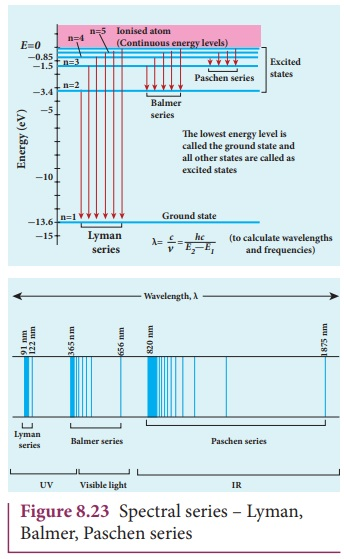Home | | Physics 12th Std | Atomic spectra

# Atomic spectra

Materials in the solid, liquid and gaseous states emit electromagnetic radiations when they are heated up and these emitted radiations usually belong to continuous spectrum.

Atomic spectra

Materials in the solid, liquid and gaseous states emit electromagnetic radiations when they are heated up and these emitted radiations usually belong to continuous spectrum. For example, when white light is examined through a spectrometer, electromagnetic radiations of all wavelengths are observed which is a continuous spectrum.

In early twentieth century, many scientists spent considerable time in understanding the characteristic radiations emitted by the atoms of individual elements exposed to a flame or electrical discharge.When they are viewed or photographed, instead of a continuous spectrum, the radiation contains of a set of discrete lines, each with characteristic wavelength. In other words, the wavelengths of the light obtained are well defined and the positions and intensities are characteristic of the element as shown in Figure 8.21.

This implies that these spectra are unique to each element and can be used to identify the element of the gas (like finger print used to identify a person) – that is, it varies from one gas to another gas. This uniqueness of line spectra of elements made the scientists to determine the composition of stars, sun and also used to identify the unknown compounds.

### Hydrogen spectrum

When the hydrogen gas enclosed in a tube is heated up, it emits electromagnetic radiations of certain sharply-defined characteristic wavelength (line spectrum), called hydrogen emission spectrum (Refer unit 5, volume 1 of +2 physics text book). The emission spectra of hydrogen are shown in Figure 8.22(a).

When any gas is heated up, the thermal energy is supplied to excite the electrons. Similarly by passing light on the atoms, electrons can be excited by absorbing photons. Once the electrons get sufficient energy as given by Bohr’s postulate (c), it absorbs energy with particular wavelength (or frequency) and jumps from its stationary state (original state) to higher energy state. Those wavelengths (or frequencies) for which the colours are not observed are seen as dark lines in the absorption spectrum as shown in Figure 8.22 (b).Since electrons in excited states have very small life time, these electrons jump back to ground state through spontaneous emission in a short duration of time (approximately 10–8 s) by emitting the radiation with same wavelength (or frequency) corresponding to the colours it absorbed (Figure 8.22 (a)). This is called emission spectroscopy.

The wavelengths of these lines can be calculated with great precision. Further, the emitted radiation contains wavelengths both lesser and greater than the visible spectrum.Notice that the spectral lines of hydrogen as shown in Figure 8.23 are grouped in separate series. In each series, the distance of separation between the consecutive wavelengths decreases from higher wavelength to the lower wavelength, and also wavelength in each series approach a limiting value known as the series limit. These series are named as Lyman series, Balmer series, Paschen series, Brackett series, Pfund series, etc. The wavelengths of these spectral lines perfectly agree with the equation derived from Bohr atom model.whereis known as wave number which is inverse of wavelength, R is known as Rydberg constant whose value is 1.09737 x 107 m-1 and m and n are positive integers such that m > n. The various spectral series are discussed below:

### (a) Lyman series

Put n = 1 and m = 2,3,4. in equation (8.18). The wave number or wavelength of spectral lines of Lyman series which lies in ultra-violet region is### (b) Balmer series

Put n = 2 and m = 3,4,5. in equation (8.18). The wave number or wavelength of spectral lines of Balmer series which lies in visible region is### (c) Paschen series

Put n = 3 and m = 4,5,6. in equation (8.18). The wave number or wavelength of spectral lines of Paschen series which lies in infra-red region (near IR) is### (d) Brackett series

Put n = 4 and m = 5,6,7. in equation (8.18). The wave number or wavelength of spectral lines of Brackett series which lies in infra-red region (middle IR) is### (e) Pfund series

Put n = 5 and m = 6,7,8. in equation (8.18). The wave number or wavelength of spectral lines of Pfund series which lies in infra-red region (far IR) isDifferent spectral series are listed in Table 8.2.### Limitations of Bohr atom model

The following are the drawbacks of Bohr atom model

(a) Bohr atom model is valid only for hydrogen atom or hydrogen like-atoms but not for complex atoms.

(b) When the spectral lines are closely examined, individual lines of hydrogen spectrum is accompanied by a number of faint lines. These are often called fine structure. This is not explained by Bohr atom model.

(c) Bohr atom model fails to explain the intensity variations in the spectral lines.

(d) The distribution of electrons in atoms is not completely explained by Bohr atom model.

Tags : Atom Models | Physics , 12th Physics : UNIT 9 : Atomic and Nuclear Physics
Study Material, Lecturing Notes, Assignment, Reference, Wiki description explanation, brief detail
12th Physics : UNIT 9 : Atomic and Nuclear Physics : Atomic spectra | Atom Models | Physics